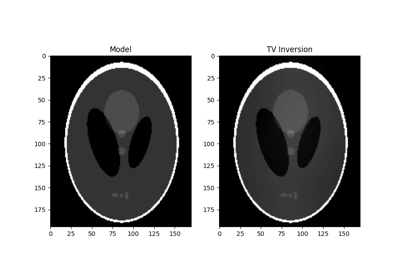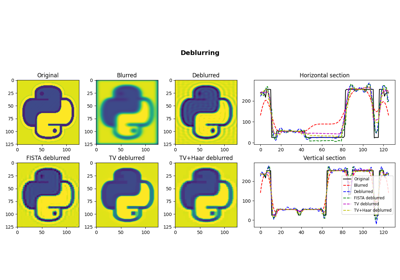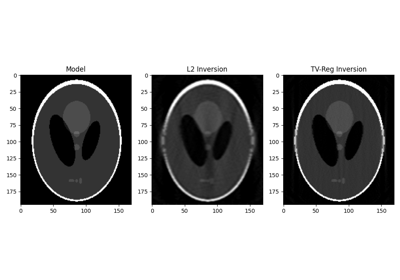# pylops.optimization.sparsity.SplitBregman¶

pylops.optimization.sparsity.SplitBregman(Op, RegsL1, data, niter_outer=3, niter_inner=5, RegsL2=None, dataregsL2=None, mu=1.0, epsRL1s=None, epsRL2s=None, tol=1e-10, tau=1.0, x0=None, restart=False, show=False, **kwargs_lsqr)[source]

Split Bregman for mixed L2-L1 norms.

Solve an unconstrained system of equations with mixed $$L^2$$ and $$L^1$$ regularization terms given the operator Op, a list of $$L^1$$ regularization terms RegsL1, and an optional list of $$L^2$$ regularization terms RegsL2.

Parameters: Op : pylops.LinearOperator Operator to invert RegsL1 : list $$L^1$$ regularization operators data : numpy.ndarray Data niter_outer : int Number of iterations of outer loop niter_inner : int Number of iterations of inner loop of first step of the Split Bregman algorithm. A small number of iterations is generally sufficient and for many applications optimal efficiency is obtained when only one iteration is performed. RegsL2 : list Additional $$L^2$$ regularization operators (if None, $$L^2$$ regularization is not added to the problem) dataregsL2 : list, optional $$L^2$$ Regularization data (must have the same number of elements of RegsL2 or equal to None to use a zero data for every regularization operator in RegsL2) mu : float, optional Data term damping epsRL1s : list $$L^1$$ Regularization dampings (must have the same number of elements as RegsL1) epsRL2s : list $$L^2$$ Regularization dampings (must have the same number of elements as RegsL2) tol : float, optional Tolerance. Stop outer iterations if difference between inverted model at subsequent iterations is smaller than tol tau : float, optional Scaling factor in the Bregman update (must be close to 1) x0 : numpy.ndarray, optional Initial guess restart : bool, optional The unconstrained inverse problem in inner loop is initialized with the initial guess (True) or with the last estimate (False) show : bool, optional Display iterations log **kwargs_lsqr Arbitrary keyword arguments for scipy.sparse.linalg.lsqr solver used to solve the first subproblem in the first step of the Split Bregman algorithm. xinv : numpy.ndarray Inverted model itn_out : int Iteration number of outer loop upon termination

Notes

Solve the following system of unconstrained, regularized equations given the operator $$\mathbf{Op}$$ and a set of mixed norm ($$L^2$$ and $$L^1$$) regularization terms $$\mathbf{R}_{2,i}$$ and $$\mathbf{R}_{1,i}$$, respectively:

$J = \frac{\mu}{2} \|\textbf{d} - \textbf{Op}\,\textbf{x} \|_2^2 + \frac{1}{2}\sum_i \epsilon_{\mathbf{R}_{2,i}} \|\mathbf{d}_{\mathbf{R}_{2,i}} - \mathbf{R}_{2,i} \textbf{x} \|_2^2 + \sum_i \epsilon_{\mathbf{R}_{1,i}} \| \mathbf{R}_{1,i} \textbf{x} \|_1$

where $$\mu$$ is the reconstruction damping, $$\epsilon_{\mathbf{R}_{2,i}}$$ are the damping factors used to weight the different $$L^2$$ regularization terms of the cost function and $$\epsilon_{\mathbf{R}_{1,i}}$$ are the damping factors used to weight the different $$L^1$$ regularization terms of the cost function.

The generalized Split-Bergman algorithm  is used to solve such cost function: the algorithm is composed of a sequence of unconstrained inverse problems and Bregman updates.

The original system of equations is initially converted into a constrained problem:

$J = \frac{\mu}{2} \|\textbf{d} - \textbf{Op}\,\textbf{x}\|_2^2 + \frac{1}{2}\sum_i \epsilon_{\mathbf{R}_{2,i}} \|\mathbf{d}_{\mathbf{R}_{2,i}} - \mathbf{R}_{2,i} \textbf{x}\|_2^2 + \sum_i \| \textbf{y}_i \|_1 \quad \text{subject to} \quad \textbf{y}_i = \mathbf{R}_{1,i} \textbf{x} \quad \forall i$

and solved as follows:

\begin{split}\DeclareMathOperator*{\argmin}{arg\,min} \begin{align} (\textbf{x}^{k+1}, \textbf{y}_i^{k+1}) = \argmin_{\mathbf{x}, \mathbf{y}_i} \|\textbf{d} - \textbf{Op}\,\textbf{x}\|_2^2 &+ \frac{1}{2}\sum_i \epsilon_{\mathbf{R}_{2,i}} \|\mathbf{d}_{\mathbf{R}_{2,i}} - \mathbf{R}_{2,i} \textbf{x}\|_2^2 \\ &+ \frac{1}{2}\sum_i \epsilon_{\mathbf{R}_{1,i}} \|\textbf{y}_i - \mathbf{R}_{1,i} \textbf{x} - \textbf{b}_i^k\|_2^2 \\ &+ \sum_i \| \textbf{y}_i \|_1 \end{align}\end{split}
$\textbf{b}_i^{k+1}=\textbf{b}_i^k + (\mathbf{R}_{1,i} \textbf{x}^{k+1} - \textbf{y}^{k+1})$

The scipy.sparse.linalg.lsqr solver and a fast shrinkage algorithm are used within a inner loop to solve the first step. The entire procedure is repeated niter_outer times until convergence.

  Goldstein T. and Osher S., “The Split Bregman Method for L1-Regularized Problems”, SIAM J. on Scientific Computing, vol. 2(2), pp. 323-343. 2008.

## Examples using pylops.optimization.sparsity.SplitBregman¶Total Variation (TV) Regularization05. Image deblurring16. CT Scan Imaging